##### Chapter 1 Review

+1
Set Details Share
created 7 years ago by teresa_gels
76 views
These notecards are formulas you must learn prior to the chapter 1 test.
Subjects:
pre calculus
Page to share:
Embed this setcancel
COPY
code changes based on your size selection
Size:
X
1

Distance Formula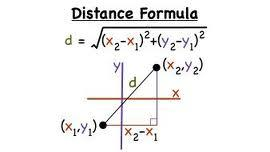2

Slope Formula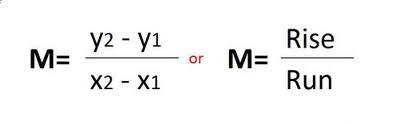3

Slope Intercept form of a line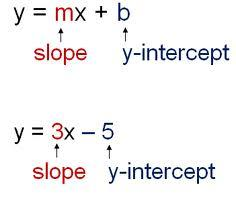4

Point Slope Form of a line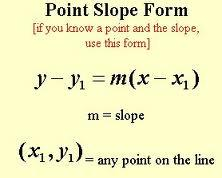5

Midpoint Formula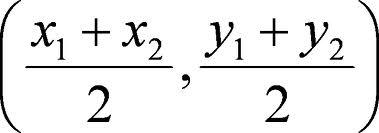6

Standard form of a line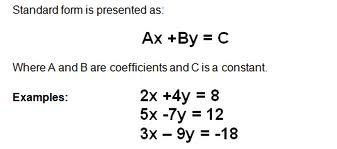7

Equation of a Circle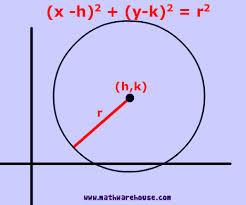8

Vertical Line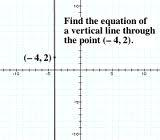9

Horizontal Line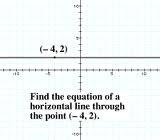10

Domain of a function

Set of all inputs possible

11

Range of a function

Set of all outputs

12

Function

All inputs have one & exactly one output (passes the vertical line test.

13

Zeros of a function (also known as roots or x-intercepts)

The value (of x) that makes the function equal to zero. [Find f(x) = 0]

14

y-intercept

The y-value where the graph of the function crosses the y-axis. [Calculate f(0)].

15

Parallel lines have ________ slopes.

Same

16

Perpendicular lines have ________ slopes

Opposite reciprocal